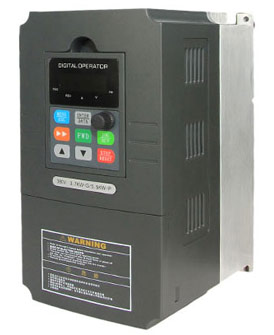Categories

# Estimate supply current to VFD from motor's torque and speed

Q:
I'm looking for ways to estimate the supply current to variable frequency drive (VFD) when an asynchronous motor is running under different torque and speed outputs.

Motor manufacturers usually provide the rated torque, rated current and rated speed of the motor, but when the motor is not running at the rated conditions, how can one estimate the supply current to the VFD from the actual speed and torque output of the motor?

A:
You should be able to estimate the power requirement using your known torques and speeds. Then just add a percentage for efficiency (say 20% for VFD and motor losses),and divide the power in kW by your line voltage to get the line current (and by root of 3 for 3-phase supply, of course). The efficiency may vary, but your torque and speed are not dependent on your motor ratings, they are a result of your load and your motor speed (~= drive frequency). So, the way I would estimate it is:

1. Power Out (kW) = Torque (N.m) x Speed (RPM) / 9.5488
2. Power In (kW) = Power Out / Efficiency
3. Line Current In = Power In / L-L Voltage In (/ sqrt(3) for 3-phase)

I'm assuming you're using an AC induction motor and that your VFD presents a unity power factor on the line side. I think maybe what you want to know is that at the motor terminals, your voltage is roughly proportional to your speed (the V/Hz ratio of your VFD output is held fairly constant), and your real/torque current is roughly proportional to your torque. But I think you're just adding an extra - somewhat unnecessary - step here, that will roughly account for the motor efficiency (side note: your motor is likely less efficient at lower speeds). Then to get the VFD's line-side current you once again divide the power by the line voltage and the VFD's efficiency.

If you manage to obtain a speed-torque-current curve for your motor, be careful as these generally apply to when the motor is being driven at the rated 50 or 60 Hz. A VFD works by shifting this curve for different speeds.

Buying VFDs on GoHz.com, 1 hp VFD, 10 hp VFD, 100 hp VFD...